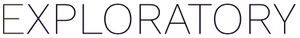# Using ifelse to mutate a column

#1

The goal here is to double the flow_mc column if the “M” (municipal) permit type is selected:

When I use this statement:

it sort of works, but it only uses the value for the first row! How can I get the output to vary by row?

Thanks in advance``````24. Create Calculation (Mutate)' step has an error. Error : Problem with `mutate()` input `calculation_1`. x `true` must be length 1 (length of `condition`), not 1040. i Input `calculation_1` is `if_else(exploratory_env\$Permit_Type == "M", flow_mc * 2, flow_mc).
``````

FYI I tried two different work arounds:

1. creating a new column for the calculation(same result) and
2. using “if_else” instead, which returned the above error.

And I can confirm that when I leave out the conditional statement, it works as expected:

Update: well maybe parameters don’t work this way? I was able to come up with a work-around that used a join and the “Replace by Setting Conditions” tool and it worked great!